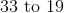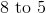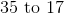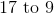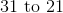# SSAT Upper Level Math : How to find the probability of an outcome

## Example Questions

← Previous 1 3 4

### Example Question #1 : Probability

A fair coin is tossed nine times. Each toss comes up heads. What is the probability that the coin will come up heads a tenth time?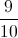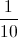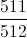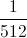Explanation:

Each flip of the coin is an independent event, and does not affect the other flips; all that matters is that the coin is fair. Because of that fact, the probability that the tenth flip comes up heads is.

### Example Question #1 : How To Find The Probability Of An Outcome

Ten balls - five green, three blue, two yellow - are placed in a hat. Two balls are drawn, one after the other, without replacement. What is the probability that at least one will be green?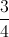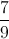Explanation:

This can more easily be solved by finding the probability that neither will be green, then subtracting it from 1.

The probability that the first ball will be not be green is five out of ten, or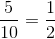.

This will leave nine balls, four not green, making the probability that the second ball will then not be green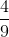.

Multiply these probabilities: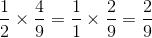This is the probability that neither ball is green; subtract this from 1: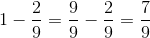### Example Question #1 : Probability

Six boxes are marked with numbers 1 to 6. Each box contains ten marbles, with the number of red marbles in each box corresponding to its number, and the remainder being white.

A fair die is rolled. The box corresponding to the roll is chosen and a marble is drawn at random from that box. What is the probability of drawing a red marble?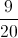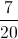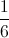Explanation:

The die is fair, making each box equally likely to be chosen; also, each marble is equally likely to be chosen after the box is chosen. This makes each marble overall equally likely to be chosen. Since there are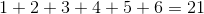red marbles out of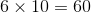marbles total, the probability of choosing a red marble is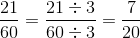### Example Question #1 : Outcomes

Six boxes are marked with numbers 1 to 6. Each box contains ten marbles, with the number of red marbles in each box corresponding to its number, and the remainder being white.

A fair die is rolled. The box corresponding to the roll is chosen and a marble is drawn at random from that box. What is the probability of drawing a white marble?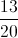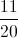Explanation:

The die is fair, making each box equally likely to be chosen; also, each marble is equally likely to be chosen after the box is chosen. This makes each marble overall equally likely to be chosen. Since there are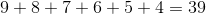white marbles out ofmarbles total, the probability of choosing a white marble is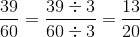### Example Question #1 : Probability

Three fair dice are rolled. What is the probability that none of them will show a 5 or a 6?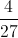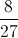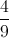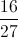Explanation:

The equivalent question is this: What is the probability that all three dice will show 1, 2, 3, or 4?

The probability that one fair die will show a 1, 2, 3, or 4 is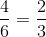, so the probability that all three will show 1, 2, 3, or 4 is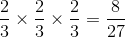.

### Example Question #1 : Outcomes

The threes from one standard deck of playing cards are added to another deck, and a card is drawn at random from that second, modified deck. What is the probability that that card will be an ace, a two, or a three?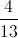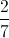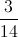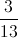Explanation:

If four more threes are added to a deck of fifty-two playing cards, then the deck will have fifty-six playing cards, including four aces, four twos, and eight threes. This makes sixteen ways to draw an ace, a two, or a three out of a possible fifty-six draws, or a probability of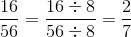.

### Example Question #1 : Probability

Ten balls - five green, three blue, two yellow - are placed in a hat. A ball is drawn, then replaced; then, a ball is drawn again. What is the probability that a blue ball will be drawn at least once?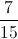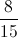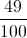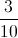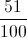Explanation:

This can more easily be solved by finding the probability that neither draw will result in a blue ball, then subtracting it from 1.

The probability that the first ball will not be blue will be seven (balls that are not blue) out of ten (balls total), or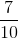. Since the ball is replaced, this is also the probability that the second will not be blue. Therefore, the probability that neither will be blue is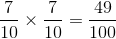The probability that at least one draw will result in a blue ball is therefore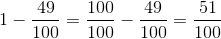.

### Example Question #31 : Data Analysis / Probablility

Two fair dice are rolled. What is the probability that at least one will show a 5 or a 6?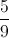Explanation:

The best way to find this probability is to find the probability that both will show a 1, 2, 3, or 4, and subtract that probability from 1.

The probability that one fair die will show a 1, 2, 3, or 4 is, so the probability that both will show 1, 2, 3, or 4 is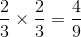.

The probability that at least one die will show a 5 or a 6 is therefore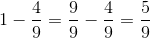.

### Example Question #2 : Probability

The tens from one standard deck of playing cards are added to another deck, and a card is drawn at random from that second, modified deck. What is the probability that that card will be an ace, a two, or a three?Explanation:

If four more tens are added to a deck of fifty-two playing cards, then the deck will have fifty-six playing cards, including four aces, four twos, and four threes. This makes twelve ways to draw an ace, a two, or a three out of a possible fifty-six draws, or a probability of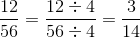.

### Example Question #2 : How To Find The Probability Of An Outcome

A card is drawn at random from a standard 52-card deck. The person drawing wins if the card he draws is a club, a king, or a queen. What are the odds against drawing a winning card?